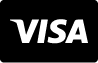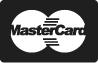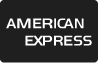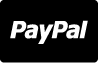Central De Ajuda
Fotos
Vetores
Vídeos
Áudios
Veja nossos planos e preços
Central De Ajuda

# Imagens - Simple subtraction problems with oranges, mathematical problems for children who study and want to learn math and calculus; learn to subtract with fruits and numbers, oranges and figures and numerical symbols

Simple subtraction problems with oranges, mathematical problems for children who study and want to learn math and calculus; learn to subtract with fruits and numbers, oranges and figures and numerical symbols
ID da imagem : 138297777
Tipo de Arquivo :
Direitos autorais :
9301 x 3829 px | 31 " x 12.76 " | 300dpi | JPG
Palavras-chave do Banco de Imagens
Mostrar mais
Show Less
Precisa de ajuda? Entre em contato com nosso atendimento
1 866 655 3733